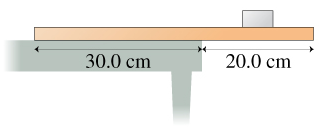# Problem: A thin 3.00 kg box rests on a 6.50 kg board that hangs over the end of a table, as shown in the figureHow far can the center of the box be from the end of the table before the board begins to tilt?

###### FREE Expert Solution

For seesaws, downward moments are the same as upward moments:

$\overline{){{\mathbf{m}}}_{{\mathbf{1}}}{{\mathbf{r}}}_{{\mathbf{1}}}{\mathbf{=}}{{\mathbf{m}}}_{{\mathbf{2}}}{{\mathbf{r}}}_{{\mathbf{2}}}\mathbf{}}$

We'll take the moments about the edge of the table.

m1 = [(30.0)/(30.0 + 20.0)](6.50) = 3.9kg

This acts at the center of 30.0cm on the left.

Thus, m1r1 = (3.9)(0.15) = 0.59 kg•m

93% (261 ratings)###### Problem Details

A thin 3.00 kg box rests on a 6.50 kg board that hangs over the end of a table, as shown in the figureHow far can the center of the box be from the end of the table before the board begins to tilt?